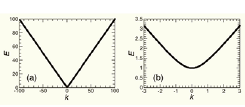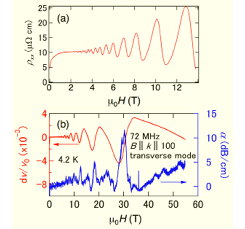Home　>　 About ISSP　>　 Publications　>　Activity Report 2018　>　Xxxx Group

## Determination of “Diracness” in PbTe

One of the hottest research subjects in condensed matter physics is so-called topological materials, in which dynamics of charge carriers are expressed by the relativistic equations of motion that are utilized in research field of high energy physics. Since the relativistic Dirac/Weyl equations show characteristic linear dispersion relation, a great number of experimental and theoretical studies have been devoted to explore the linear dispersion as schematically shown in Fig. 1(a), which is distinguished from the parabolic one shown in Fig. 1(b). This parabolic dispersion, however, is merely the expanded view of the trace in Fig. 1(a) at around the origin. Therefore, argument based only on the appearance of the dispersion is sensuous and insufficient to quantitatively distinguish the Dirac/Weyl system from the others. As the quantitative indicator that characterizes similarity of a system with the ideal Dirac system, which is called as “Diracness” in the following, we focus on the ratio between Zeeman and cyclotron energies (ZC ratio). Recent theoretical studies revealed that this ZC ratio becomes unity for the ideal Dirac system [1,2]. Relative magnitude of the ZC ratio and unity is predicted to invert when topology of a material changes from trivial to non-trivial.Fig. 1. (a) Schematic dispersion relation of kinetic motion of particles that is expressed by relativistic Dirac equation, which shows characteristic linear dispersion in wide range of the k-space. (b) Expanded view the same dispersion relation with that in (a), which appears as parabolic in this region.Fig. 2. (a) Transverse magnetoresistance and (b) ultrasound attenuation in single crystals of PbTe. Quantum oscillations are clearly resolved in both experiments.

To experimentally determine the ZC ratio, we studied quantum oscillations in single crystals of PbTe through measurements of magnetoresistance (Fig. 2(a)), magnetization, and ultrasound attenuations (Fig. 2(b)) in DC and pulsed high magnetic fields . By measuring quantum oscillations up to the quantum limit state and comparing the results with the standard Lifshitz-Kosevich formula , we determined the ZC ratio of PbTe as 0.52. In this analysis, we clarified that when the ZC ratio becomes larger than one half, peak-dip relation in the quantum oscillation will be reversed, which will appear as the phase shift in the Landau level fan diagram. The results demonstrate that observation of spin-split quantum oscillations is crucially important to discuss the origin of the phase shift.

##### Authors
• K. Akiba, A. Miyake, H. Sakaia,b, K. Katayamaa, H. Murakawaa, N. Hanasakia, S. Takaokaa, Y. Nakanishc, M. Yoshizawac, and M. Tokunaga
• aOsaka University
• bJST-PRESTO
• cIwate University